# How to Evaluate Models

#### 2018-02-08

A big part of the purpose of idealstan is to give people different options in fitting ideal point models to diverse data. Along with that, idealstan makes use of Bayesian model evaluation a la loo and also can analyze the posterior predictive distribution using bayesplot. loo is an approximation of the predictive error of a Bayesian model via leave-one-out cross-validation (LOO-CV). True LOO-CV on Bayesian models is computationally prohibitive because it involves estimating a new model for each data point. For IRT models incorporating thousands or even millions of observations, this is practically infeasible.

bayesplot allows us to analyze the data we used to estimate the model compared to data produced by the model, or what is called the posterior predictive distribution. This is very useful as a general summary of model fit to see whether there are values of the outcome that we are over or under predicting.

idealstan implements functions for each ideal point model that calculate the log-posterior probability of the data, which is the necessary input to use loo’s model evaluation features. This vignette demonstrates the basic usage.

We first begin by simulating data for a standard IRT 2-PL ideal point model but with strategically missing data:

irt_2pl <- id_sim_gen(ordinal=FALSE,absence=TRUE)

We can then fit two ideal point models to the same data, one that uses constraints for identification and the other that pins two of the persons to arbitrary (and incorrect) values.

# Because of CRAN limitations, only using 2 cores & 2 chains
irt_2pl_correct <- id_estimate(idealdata=irt_2pl,
model_type=2,
restrict_ind_high = sort(irt_2pl@simul_data$true_reg_discrim, decreasing=TRUE, index=TRUE)$ix[1:3],
restrict_ind_low = sort(irt_2pl@simul_data$true_reg_discrim, decreasing=FALSE, index=TRUE)$ix[1:3],
restrict_params = 'discrim_reg',
restrict_type = 'constrain_twoway',
fixtype='constrained',
ncores=2,
nchains=2)

irt_2pl_incorrect <- id_estimate(idealdata=irt_2pl,
model_type=2,
restrict_ind_high = c(1,2),
restrict_params = 'person',
pin_vals=c(-1,1.5),
fixtype='pinned',
ncores=2,
nchains=2)

The first thing we want to check with any MCMC model is convergence. An easy way to check is by looking at the Rhat distributions. If all these values are below 1.1, then we have good reason to believe that the model converged, and we can get these distributions with the id_plot_rhats function:

id_plot_rhats(irt_2pl_correct)
## stat_bin() using bins = 30. Pick better value with binwidth.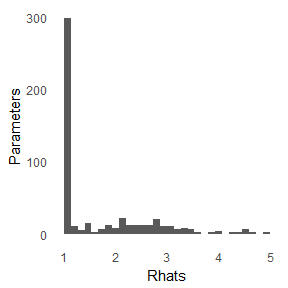id_plot_rhats(irt_2pl_incorrect)
## stat_bin() using bins = 30. Pick better value with binwidth.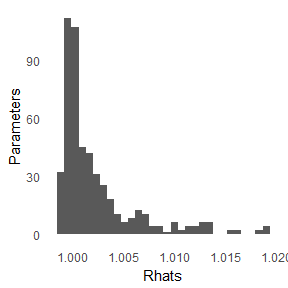Only the first model seems to have converged–at this point we should go back and see if the model is miss-specified or there is something wrong with the data. But for the sake of illustration, we will look at other diagnostics. We can also examine whether 1) the models are able to replicate the data they were fitted on accurately and 2) overall measures of model fit.

We can first look at how well the model reproduces the data, which is called the posterior predictive distribution. We can obtain these distributions using the id_post_pred function:

post_correct <- id_post_pred(irt_2pl_correct)
##  "Processing posterior replications for 1000 scores using 100 posterior samples out of a total of 2000 samples."
post_incorrect <- id_post_pred(irt_2pl_incorrect)
##  "Processing posterior replications for 1000 scores using 100 posterior samples out of a total of 2000 samples."

What we can do is the use a wrapper around the bayesplot package called id_plot_ppc to see how well these models replicate their own data:

id_plot_ppc(irt_2pl_correct,ppc_pred=post_correct)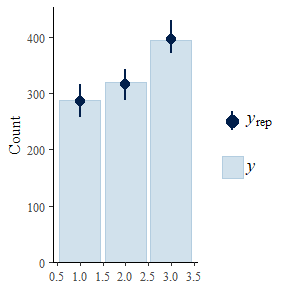id_plot_ppc(irt_2pl_incorrect,ppc_pred=post_incorrect)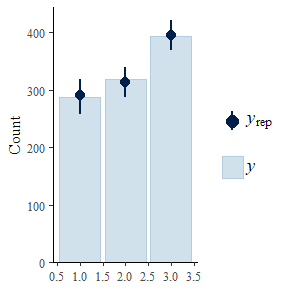Even though the incorrect model didn’t converge, it can still replicate the posterior data quite well. We can also look at particular persons or items to see how well the models predict those persons or items. For example, let’s look at the first two persons in the simulated data for which we fixed that person’s value to an arbitrary numbe:

id_plot_ppc(irt_2pl_incorrect,ppc_pred=post_incorrect,person=c(1,2))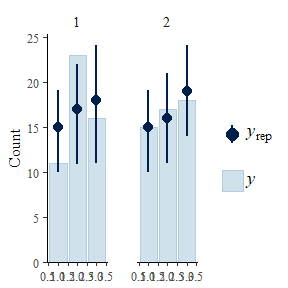Finally, we can turn to summary measures of model fit that also allow us to compare models directly to each other (if they were fit on the same data). To do so, I first employ the id_log_lik function to generate log-likelihood values for each of these models:

log_lik_irt_2pl_correct <- id_log_lik(irt_2pl_correct)
##  "Processing posterior replications for 1000 scores using 100 posterior samples out of a total of 2000 samples."
log_like_irt_2pl_incorrect <- id_log_lik(irt_2pl_incorrect)
##  "Processing posterior replications for 1000 scores using 100 posterior samples out of a total of 2000 samples."

With this calculation can examine the models’ loo values, which shows the relative predictive performance of the model to the data. Overall, model performance seems quite good, as the Pareto k values show that there are only a few dozen observations in the dataset that aren’t well predicted. The LOO-IC, or the leave-one-out information criterion (think AIC or BIC), are also similar for the two models, although the correct model does have a lower (i.e., better) LOO-IC.

loo(log_lik_irt_2pl_correct,cores=2)
## Warning: Some Pareto k diagnostic values are too high. See help('pareto-k-
## diagnostic') for details.
## Computed from 100 by 1000 log-likelihood matrix
##
##          Estimate   SE
## elpd_loo  -1070.7 21.5
## p_loo       103.3  5.7
## looic      2141.3 43.1
##
## Pareto k diagnostic values:
##                          Count  Pct
## (-Inf, 0.5]   (good)     816   81.6%
##  (0.5, 0.7]   (ok)        96    9.6%
##    (0.7, 1]   (bad)       68    6.8%
##    (1, Inf)   (very bad)  20    2.0%
## See help('pareto-k-diagnostic') for details.
loo(log_like_irt_2pl_incorrect,cores=2)
## Warning: Some Pareto k diagnostic values are too high. See help('pareto-k-
## diagnostic') for details.
## Computed from 100 by 1000 log-likelihood matrix
##
##          Estimate   SE
## elpd_loo  -1058.4 21.7
## p_loo       100.3  5.9
## looic      2116.9 43.4
##
## Pareto k diagnostic values:
##                          Count  Pct
## (-Inf, 0.5]   (good)     765   76.5%
##  (0.5, 0.7]   (ok)       126   12.6%
##    (0.7, 1]   (bad)       78    7.8%
##    (1, Inf)   (very bad)  31    3.1%
## See help('pareto-k-diagnostic') for details.

We can also compare the LOOIC of the two models explicitly using a second loo function that will even give us a confidence interval around the difference. If the difference is negative, then the first (correct) model has higher predictive accuracy:

compare(loo(log_lik_irt_2pl_correct,cores=2),
loo(log_like_irt_2pl_incorrect,cores=2))
## Warning: Some Pareto k diagnostic values are too high. See help('pareto-k-
## diagnostic') for details.

## Warning: Some Pareto k diagnostic values are too high. See help('pareto-k-
## diagnostic') for details.
## elpd_diff        se
##      12.2      30.7